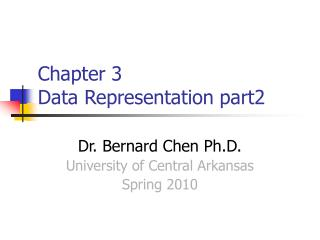DownloadDownload PresentationChapter 3 Data Representation part2

# Chapter 3 Data Representation part2

Download Presentation## Chapter 3 Data Representation part2

- - - - - - - - - - - - - - - - - - - - - - - - - - - E N D - - - - - - - - - - - - - - - - - - - - - - - - - - -
##### Presentation Transcript

1. Chapter 3 Data Representation part2 Dr. Bernard Chen Ph.D. University of Central Arkansas Spring 2010

2. Outline • Subtraction of Unsigned Numbers using r’s complement • How To Represent Signed Numbers • Floating-Point Representation

3. Subtraction of Unsigned Numbers using r’s complement • (1) if M  N, ignore the carry without taking complement of sum. • (2) if M < N, take the r’s complement of sum and place negative sign in front of sum. The answer is negative.

4. Example 1 (Decimal unsigned numbers), perform the subtraction 72532 - 13250 = 59282. M > N : “Case 1” “Do not take complement of sum and discard carry” The 10’s complement of 13250 is 86750. Therefore: M = 72532 10’s complement of N =+86750 Sum= 159282 Discard end carry 105= - 100000 Answer = 59282 no complement

5. Example 2; Now consider an example with M <N. The subtraction 13250 - 72532 produces negative 59282. Using the procedure with complements, we have M = 13250 10’s complement of N = +27468 Sum = 40718 Take 10’s complement of Sum = 100000 -40718 The number is : 59282 Place negative sign in front of the number: -59282

6. Subtract by Summation Subtraction with complement is done with binary numbers in a similar way. Using two binary numbers X=1010100 and Y=1000011 We perform X-Y and Y-X

7. X-Y X= 1010100 2’s com. of Y= 0111101 Sum= 10010001 Answer= 0010001

8. Y-X Y= 1000011 2’s com. of X= 0101100 Sum= 1101111 There’s no end carry: answer is negative --- 0010001 (2’s complement of 1101111)

9. Outline • Subtraction of Unsigned Numbers using r’s complement • How To Represent Signed Numbers • Floating-Point Representation

10. How To Represent Signed Numbers Plus and minus signs used for decimal numbers: 25 (or +25), -16, etc. For computers, it is desirable to represent everything as bits. Three types of signed binary number representations: signed magnitude, 1’s complement, and 2’s complement

11. 1. signed magnitude In each case:left-most bit indicates sign: positive (0) or negative (1). 000011002= 1210 100011002= -1210 Sign bit Sign bit Magnitude Magnitude Consider 1.signed magnitude:

12. 2. One’s Complement Representation The one’s complement of a binary number involves inverting all bits. To find negative of 1’s complement number take the 1’s complement of whole number including the sign bit. 000011002 = 1210 111100112= -1210 Sign bit Sign bit Magnitude 1’complement

13. 3. Two’s Complement Representation The two’s complement of a binary number involves inverting all bits and adding 1. To find the negative of a signed number take the 2’s the 2’s complement of the positive number including the sign bit. 000011002= 1210 111101002 = -1210 Sign bit Sign bit Magnitude 2’s complement

14. Sign addition in 2’s complement The rule for addition is add the two numbers, including their sign bits, and discard any carry out of the sign (leftmost) bit position. Numerical examples for addition are shown below. Example: + 6 00000110 - 6 11111010+1300001101+1300001101 +19 00010011 +7 00000111 +6 00000110 -6 11111010 -1311110011 -1311110011 -7 11111001 -19 11101101 In each of the four cases, the operation performed is always addition, including the sign bits. Only one rule for addition, no separate treatment of subtraction. Negative numbers are always represented in 2’s complement.

15. Arithmetic Subtraction A subtraction operation can be changed to an addition operation if the sign of the subtrahend is changed. (±A) - (+B) = (±A) + (-B) (±A) - (-B) = (±A) + (+B)

16. Arithmetic Subtraction Consider the subtraction of (-6) - (-13) = +7. In binary with eight bits this is written as 11111010 - 11110011. The subtraction is changed to addition by taking the 2’s complement of the subtrahend (-13) to give (+13). In binary this is 11111010 + 00001101 = 100000111. Removing the end carry, we obtain the correct answer 00000111 (+ 7).

17. Overflow Overflow example: +70 0 1000110 -70 1 0111010 +80 0 1010000 -80 1 0110000 = +150 1 0010110 =-150 0 1101010

18. Overflow An overflow cannot occur after an addition if one number is positive and the other is negative, since adding a positive number to a negative number produces a result that is smaller than the larger of the two original numbers. An overflow may occur if the two numbers added are both either positive or negative.

19. Outline • Subtraction of Unsigned Numbers using r’s complement • How To Represent Signed Numbers • Floating-Point Representation

20. Floating-Point Representation + 6132.789 is represented in floating-point with a fraction and an exponent as follows: Fraction Exponent +0.6132789 +04 Scientific notation : + 0.6132789  10+4

21. Floating-Point Representation 32-bit floating point format. Leftmost bit = sign bit (0 positive or 1 negative). Exponent in the next 8 bits. Use a biased representation. Final portion of word (23 bits in this example) is the significand (sometimes called mantissa).

22. Example Convert the following number;37.75 into floating point format to fit in 32 bit register. Convert the number from decimal into binary 100101.11 Normalize all digits including the fraction to determine the exponent. 1.0010111 x 25 sign sign EXP EXP Significant

23. Outline • Subtraction of Unsigned Numbers using r’s complement • How To Represent Signed Numbers • Floating-Point Representation